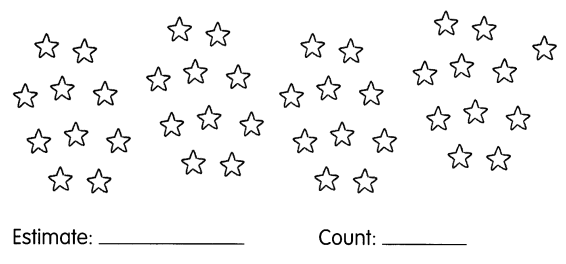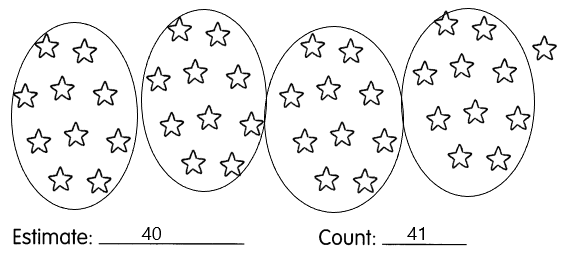# Math in Focus Grade 1 Chapter 16 Practice 1 Answer Key Counting to 100

Go through the Math in Focus Grade 1 Workbook Answer Key Chapter 16 Practice 1 Counting to 100 to finish your assignments.

## Math in Focus Grade 1 Chapter 16 Practice 1 Answer Key Counting to 100

Count in tens and ones.

Fill in the blanks.

Example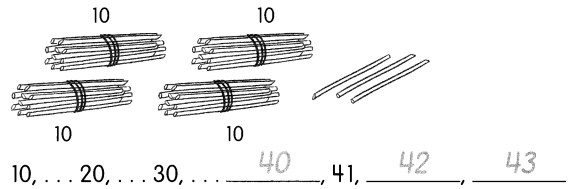Question 1.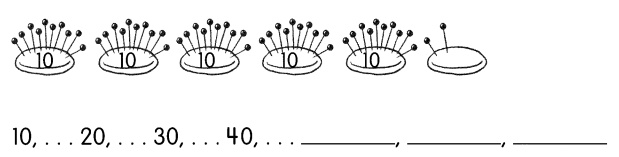10,20,30,40,50,51,52.

Explanation:
In the above image, we can see that there are 5 tens and 2 ones which is 52.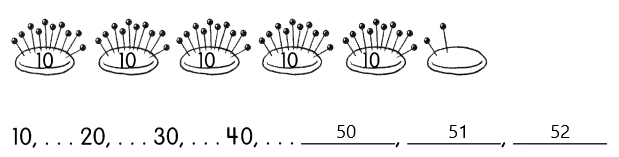Question 2.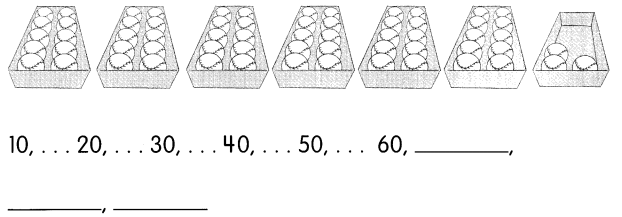10,20,30,40,50,60,61,62,63.

Explanation:
In the above image, we can see that there are 6 tens and 3 ones which is 63.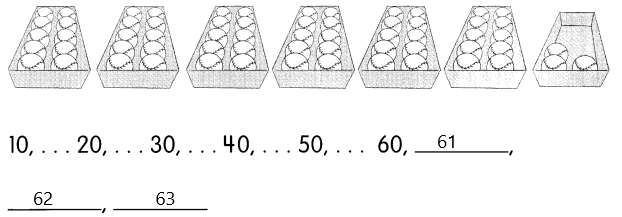Question 3.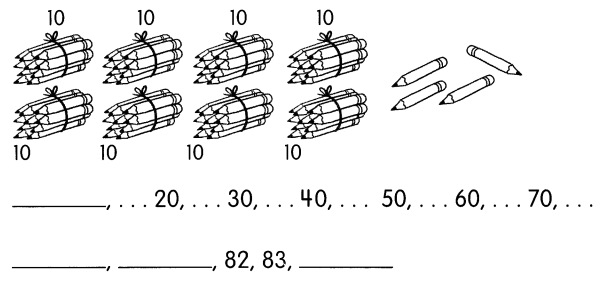10,20,30,40,50,60,70,80,81,82,83,84.

Explanation:
In the above image, we can see that there are 8 tens and 4 ones which is 84.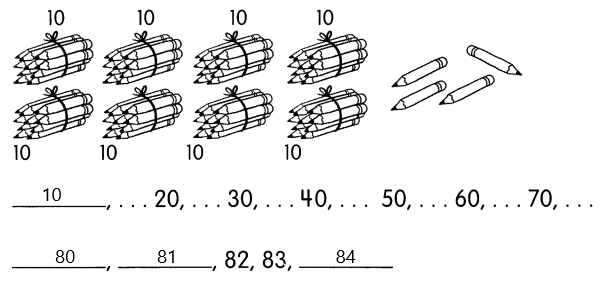Write the number.

Question 4.
forty-nine _____
forty-nine – 45.

Explanation:
Given that forty-nine, so in numbers, it will be 45.

Question 5.
sixty-eight _____
sixty-eight – 68.

Explanation:
Given that sixty-eight, so in numbers, it will be 68.

Question 6.
ninety-five _____
ninety-five – 95.

Explanation:
Given that ninety-five, so in numbers, it will be 95.

Question 7.
eighty-seven _____
eighty-seven – 87.

Explanation:
Given that eighty-seven, so in numbers, it will be 87.

Question 8.
fifty-six _____
fifty-six – 56.

Explanation:
Given that fifty-six, so in numbers, it will be 56.

Question 9.
seventy-three _____
seventy-three – 73.

Explanation:
Given that seventy-three, so in numbers, it will be 73.

Question 10.
ninety-two _____
ninety-two – 92.

Explanation:
Given that ninety-two, so in numbers, it will be 92.

Match the number to the words.

Question 11.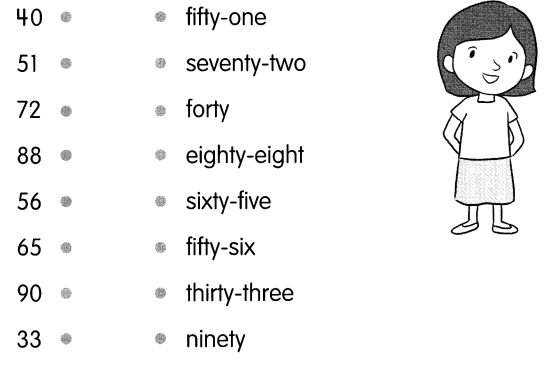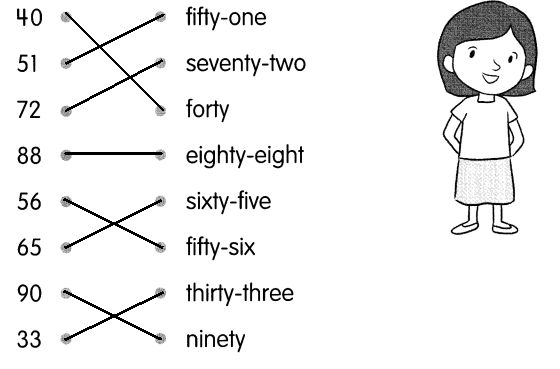Find the missing numbers.

Question 12.
60 and 4 make _____
60 and 4 make 64.

Explanation:
Given that 60 and 4 make 64.

Question 13.
5 and 70 make _____
5 and 70 make 75.

Explanation:
Given that 5 and 70 make 75.

Question 14.
50 and ____ make 53.
50 and 3 make 53.

Explanation:
Here, 53 makes 50 and 3.

Question 15.
____ and 4 make 84.
80 and 4 make 84.

Explanation:
Here, 84 makes 80 and 4.

Question 16.
40 + 5 = ____
40 + 5 = 45.

Explanation:
Given that 40+5 makes 45.

Question 17.
___ + 80 = 88
8 + 80 = 88.

Explanation:
Here, 88 makes 8 + 80.

Circle a group of 10. Estimate how many there are. Then count.

Question 18.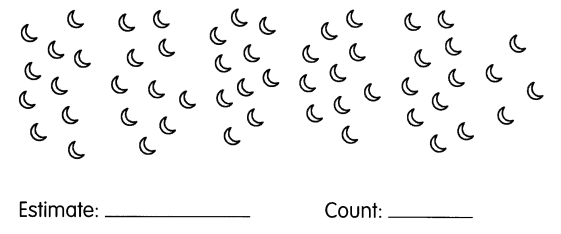The actual count is 50 and the estimated is 55.

Explanation:
In the image, we can see 5 groups with 10 half-moons. So the estimation will be 55 and the count is 50.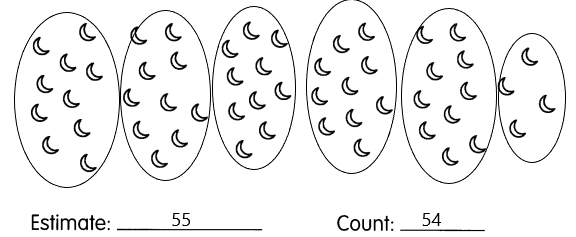Question 19.